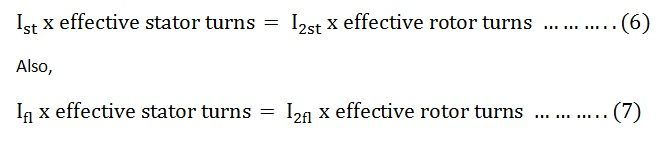# Direct On Line Starter

Direct On Line Starter method is a common method of starting of Cage Induction Motor. The motor is connected through a starter across the full supply voltage. The Direct On Line Starter Method figure is shown below. It consists a coil operated contactor C controlled by start and stop push button as shown in the connection diagram below:The buttons which may be installed in a convenient place away from the starter. The start button is held open by a spring. On pressing the START pushbutton S1, the contactor C is energised from two line conductors L1 and L2.

The three main contacts M and the auxiliary contact A are closed. The terminals a and b are short-circuited. The motor is then connected to the supply mains. The S1 button moves back under the spring action as soon as the pressure is released. The coil C remains energised through ab.

Thus, the main contact M remains closed, and the motor continues to get supplies. Therefore, contact A is known as Hold-On-Contact. The stop button S2 is normally held closed by the spring. If the S2 button is pressed to STOP the motor, the supply through the contactor coil C is disconnected. As the coil C is de-energized, the main contacts M and the auxiliary contact A are opened. The supply to the motor is disconnected, and the motor is stopped.

### Undervoltage Protection

When the voltage falls below a certain value or when the supply fails or disrupted during the operation of the motor, the coil C is de-energized. Hence, the motor is disconnected from the supplyOverload Protection

The motor is overloaded, one or all the overload coils (O.L.C) are energised. The normally closed contact D is opened, and the contactor coil C is de-energized to disconnect the supply from the motor. Fuses are provided in the circuit for short circuit protection.

In direct on line starting the starting current may be as large as ten times the full load current, and the starting torque is equal to full load torque. Such a large starting current produces an excessive voltage drop in the line which supplies power to the motor.

## Theory of Direct On Line Starting of Induction Motor

Let,

• Ist be the starting current drawn from the supply mains per phase.
• Ifl is the full load current drawn from the supply mains per phase.
• Ʈest is the starting torque.
• Sfl is the slip at full load.

As we know that the rotor copper loss = s x rotor inputAt starting, s = 1, I2 = I2st, Ʈe = Ʈest

At full loads = sfl, I2 = I2fl, Ʈe = ƮeflIf the no load current is neglectedEquating the above equation (6) and (7), we getFrom the equation (5) and (8) we getIf V1 is the stator voltage per phase equivalent

Ze10 is the standstill impedance per phase of the motor referred to the stator then the current at the starting is given by the equation shown below.The starting current is equal to the short circuit current.

Combining equation (9) and (10) we getDirect on line starter method is the cheapest and the simplest method of the starting of the induction motor.